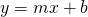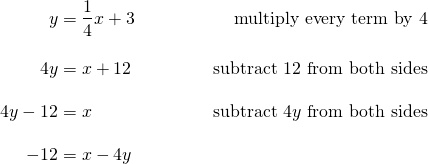# Linear Functions: ax+by=c

The general form for a line iswhereandare integers.

How does it work? Video

Using either the slope intercept form () or the general form () we can calculate all the critical values of a straight line. These are: theintercept, theintercept and the slope.

Example 1: converttoform:Example 2: convertto general form:# Finding the y intercept

The y intercept is the point where the line cuts the y-axis. The x coordinate is zero. To find the y intercept, we substitutefor.

Example 3: Find the y intercept of the line# Finding the x intercept

The x intercept is the point that is on the line and on the x axis. The y coordinate is zero. To find the x intercept, we substitutefor.

Example 4: Find the x intercept of the line# Calculations

Example: Video (3:31 mins)

5 Examples for this applet: Video (16 mins)

Examples for this applet on a pdf: Examples x y intercepts slope general form

Calculate the x and y intercepts and the slope. Rewrite the equation in the alternate form. Use fractions or terminating decimals, don’t round decimals.